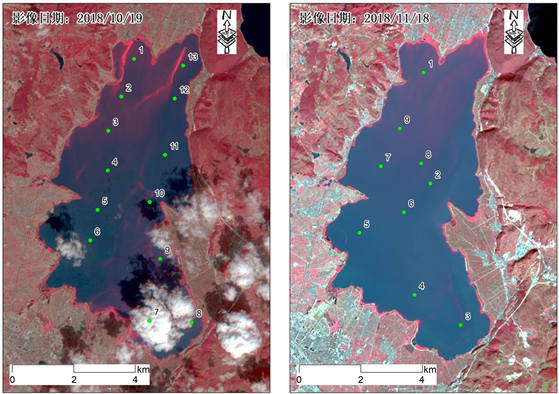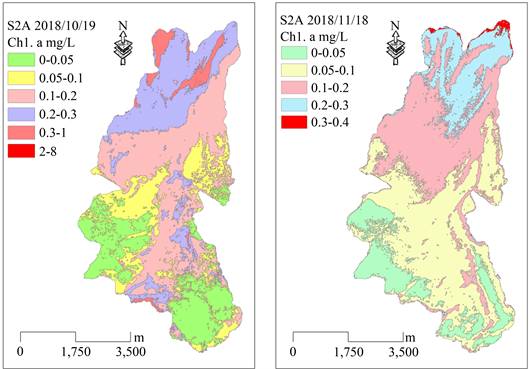# 基于Sentinel-2卫星的星云湖叶绿素a遥感估算研究Remote Estimation of Chlorophyll-a in Xingyun Lake by Sentinel-2 Satellite

• 全文下载: PDF(743KB)    PP.20-31   DOI: 10.12677/AEP.2020.101003
• 下载量: 59  浏览量: 95   国家科技经费支持

Sentinel-2A satellite was used to establish chlorophyll-a remote sensing estimation models for the Xingyun Lake in this study. The correlation between the spectral reflectivity of each band and the concentration of chlorophyll-a was analyzed by the concurrent observation. According to DVI, RVI, NDVI, TBM and MCI algorithms, a combination of different sensitive bands and algorithms is used to set up 21 models. Then the modeling dataset is divided into all datasets, high and low concentration datasets. Three data sets are substituted into 21 models to establish 63 regression equations. The modeling and validation effects of these regression equations are analyzed. The remote sensing estimation of chlorophyll-a concentration in Xingyun Lake is carried out by using different models in different chlorophyll-a concentration ranges of Xingyun Lake. The result shows that: 1) The spectral reflectivity of the 4 Red-Edge bands and Near-Infrared band of the Sentinel-2 satellite is strongly positive related to the concentration of chlorophyll-a. When the concentration of chlo-rophyll-a was higher than that of 0.1 mg/L, the best model is that the rRMSE and NMAE of RVI1H, were 4.01% and 3.95%, respectively. When the concentration of chlorophyll-a is lower than 0.1 mg/L, the best model is that the rRMSE and NMAE of NDVI1L are 25.95% and 19.32%, respectively. TBM1L model is more suitable to calculate the average value of the whole lake, and its MNB is −0.57%. 2) The regression equation of modeling has high determination coefficient, which can only show that the linearity of the modeling data set is better, but whether the model is applicable or not is mainly based on the error of the verification data.

1. 引言

Sentinel-2 (哨兵2)卫星是欧盟“全球环境与安全监测”计划的第二颗卫星，由两颗卫星组成(A星和B星)，携带一枚多光谱成像仪MSI，拥有13个光谱波段，10 m空间分辨率，双星重访周期达到5d，目前全世界在轨的可以公开获取数据的多光谱卫星中，Sentinel-2是空间分辨率和光谱分辨率最高的。采用哨兵2对星云湖进行遥感监测，可以获得34万个像元，空间解析度远比MODIS高 。

2. 研究方法

2.1. 采样点布设Figure 1. Sampling point layout and the Sentinel-2A satellite image at pseudo-color

2.2. 水样叶绿素a的测定方法

$\text{Chl}\text{.a}=27.9{V}_{乙醇}\left[\left({E}_{665}-{E}_{750}\right)-\left({A}_{665}-{A}_{750}\right)\right]/{V}_{样品}$ (1)

2.3. 卫星数据

2.4. 波段敏感性分析及叶绿素a估算模型的建立

$\text{RVI}={R}_{rs}\left({\lambda }_{2}\right)/{R}_{rs}\left({\lambda }_{1}\right)H$ (2)

$\text{DVI}={R}_{rs}\left({\lambda }_{2}\right)-{R}_{rs}\left({\lambda }_{1}\right)$ (3)

$\text{NDVI}=\frac{\left({R}_{\text{rs}}\left({\lambda }_{2}\right)-{R}_{rs}\left({\lambda }_{1}\right)\right)}{\left({R}_{\text{rs}}\left({\lambda }_{2}\right)+{R}_{rs}\left({\lambda }_{1}\right)\right)}$ (4)

$\text{TBM}=\left[{R}_{rs}{\left({\lambda }_{1}\right)}^{-1}-{R}_{rs}{\left({\lambda }_{2}\right)}^{-1}\right]{R}_{rs}\left({\lambda }_{3}\right)$ (5)

$\text{MCI}={R}_{rs}\left({\lambda }_{2}\right)-{R}_{rs}\left({\lambda }_{1}\right)-\left[\frac{\left({\lambda }_{2}-{\lambda }_{1}\right)}{\left({\lambda }_{3}-{\lambda }_{1}\right)}\left({R}_{rs}\left({\lambda }_{3}\right)-{R}_{rs}\left({\lambda }_{1}\right)\right)\right]$ (6)Table 1. Monitoring data of chlorophyll-a in Xingyun Lake (mg/L)

① All dataset; ② Data with a concentration greater than 0.1 mg/L of chlorophyll-a is a high concentration dataset; ③ Data with a concentration lower than 0.1 mg/L of chlorophyll-a is a low concentration dataset.

① 全部数据集；② 叶绿素a浓度大于0.1 mg/L的数据为高浓度数据集；③ 叶绿素a浓度小于0.1 mg/L的数据集为低浓度数据集。

2.5. 误差的估算

$\text{RMSE}=\sqrt{\frac{\underset{i=1}{\overset{N}{\sum }}{\left({X}_{\text{esti},i}-{X}_{\text{meas},i}\right)}^{2}}{N-1}}$ (7)

$\text{rRMSE}=\text{RMSE}/{\text{Mean}}_{\text{meas}}$ (8)

NRMS表示结果的相对随机不确定性，它代表 ${\epsilon }_{i}$ 的标准差，计算公式为：

$\text{NRMS}=\text{stdev}\left({\epsilon }_{i}\right)%$ (9)

${\epsilon }_{i}$ 是测量值与估算值之差所占实测值的百分比，计算公式如下：

${\epsilon }_{i}=\left(\left({X}_{\text{esti},i}-{X}_{\text{meas},i}\right)/{X}_{\text{meas},i}\right)×100$ (10)

MNB表示估算的平均偏差，它代表 ${\epsilon }_{i}$ 的平均值；NMAE表示估算的平均绝对误差，它代表 ${\epsilon }_{i}$ 的绝对值的平均值，计算公式如下：

$\text{MNB}\left(%\right)=\text{mean}\left({\epsilon }_{i}\right)$ (11)

$\text{NMAE}\left(%\right)=\text{mean}\left(|{\epsilon }_{i}|\right)$ (12)

3. 研究结果与讨论

3.1. 叶绿素a浓度测定结果与波段敏感性分析Table 2. Pearson correlation analysis result

*At level 0.05 (double tail)，**At level 0.01 (double tail)，the correlation was significant.

*在0.05级别(双尾)，**在0.01级别(双尾)，相关性显著。

3.2. 模型的构建和验证Table 3. Estimation and Verification results of chlorophyll-a model (All dataset)

Table 4. Estimation and Verification results of chlorophyll-a model (High dataset)Table 5. Estimation and Verification results of chlorophyll-a model (Low dataset)

3.3. 模型应用Figure 2. Remote estimation of chlorophyll-a in the Sentinel-2A satellite at the Xingyun LakeTable 6. The results of remote estimation chlorophyll-a concentration at Xingyun Lake (mg/L)

4. 结论

1) 哨兵2卫星4个红边波段和近红外波段反射率均与叶绿素a浓度呈强烈正相关，叶绿素a浓度高于0.1 mg/L时，采用RVI1H模型，误差最低，其rRMSE和NMAE分别为4.01%和3.95%。叶绿素a浓度低于0.1 mg/L时，采用NDVI1L模型进行估算，rRMSE为25.95%，NMAE为19.32%，MNB为−13.3%，采用TBM1L模型估算时，MNB为−0.57%，说明模拟值与实测值之间正负偏离抵消较多，比较适用于计算全湖的平均值。

2) 基于浓度分区对星云湖叶绿素a进行估算，2018年10月19日估算的叶绿素a浓度大于同年11月18日的估算结果，这个结论与标准假彩色影像的感官结果类似。

3) 低浓度叶绿素a估算模型误差较高浓度模型的误差大，主要原因是本研究基于两次不同时间的星地同步和准同步观测来建模和验证，不同时间的卫星数据，由于大气状况不同，大气校正也并不是完美地消除所有大气的影响，相同的地物在两次不同时相的影像中的反射率值是有细微的差别的，而不同批次的采样和实验也会带来一定的误差，气象因子方面，风的作用会改变浮藻的位置，而低浓度范围的叶绿素a估算精度更容易受到这些误差的影响。在今后的研究当中需要积累更多的星地同步观测数据，同时也要加强对星云湖中其它光学敏感物质的监测研究，排除各种干扰物质的影响，进一步提高叶绿素a的估算精度。

4) 建模的时候得到的回归方程的决定系数高，只能说明建模数据集的线性较好，但是模型是否适用，主要依据还是验证数据的误差。

NOTES

*通讯作者。

  O’Reilly, J.E. and Werdell, P.J. (2019) Chlorophyll Algorithms for Ocean Color Sensors: OC4, OC5 & OC6. Remote Sensing of Environment, 229, 32-47. https://doi.org/10.1016/j.rse.2019.04.021  Gupta, R.K., Prasad, S., Nadham, T.S.V., et al. (1993) Relative Sensitivity of District Mean RVI and NDVI over an Agrometeorological Zone. Advances in Space Research, 13, 261-264. https://doi.org/10.1016/0273-1177(93)90555-P  Zhang, J., Li, M., Sun, Z., et al. (2018) Chlorophyll Content Detection of Field Maize Using RGB-NIR Camera. IFAC-PapersOnLine, 51, 700-705. https://doi.org/10.1016/j.ifacol.2018.08.114  Giorgio, D.O. and Gitelson, A.A. (2005) Effect of Bio-Optical Parameter Variability on the Remote Estimation of Chlorophyll-a Concentration in Turbid Productive Waters: Experimental Results. Applied Optics, 44, 412-422. https://doi.org/10.1364/AO.44.000412  Song, K., Lin, L., Tedesco, L.P., et al. (2013) Remote Estimation of Chlorophyll-a in Turbid Inland Waters: Three-Band Model versus GA-PLS Model. Remote Sensing of Environment, 136, 342-357. https://doi.org/10.1016/j.rse.2013.05.017  刘阁, 李云梅, 吕恒, 等. 基于MERIS影像的洪泽湖叶绿素a浓度时空变化规律分析[J]. 环境科学, 2017, 38(9): 3645-3656.  周琳, 马荣华, 段洪涛, 等. 浑浊II类水体叶绿素a浓度遥感反演(I): 模型的选择[J]. 红外与毫米波学报, 2011, 30(6): 531-536.  Xu, J.P., Fang, L., Bai, Z., et al. (2008) Improved Conceptual Three-Band Model for Chlorophyll-a Retrieval in Inland Case-II Waters. Proceedings of SPIE—The International Society for Optical Engineering, Volume 7145, 71451L. https://doi.org/10.1117/12.813039  Matsushita, B., et al. (2015) A Hybrid Algorithm for Estimating the Chlorophyll-a Concentration across Different Trophic States in Asian Inland Waters. ISPRS Journal of Photogrammetry and Remote Sensing, 102, 28-37. https://doi.org/10.1016/j.isprsjprs.2014.12.022  Christopherson, J.B., Ramaseri Chandra, S.N. and Quanbeck, J.Q. (2019) Joint Agency Commercial Imagery Evaluation—Land Remote Sensing Satellite Compendium. 208. https://doi.org/10.3133/cir1455  杨国范, 阎孟冬, 殷飞. 清河水库叶绿素a浓度反演模型研究[J]. 遥感信息, 2016, 31(5): 74-78.  杨硕, 王世新, 周艺, 等. 叶绿素反演三波段模型的多时相应用[J]. 遥感信息, 2010(5): 98-104.  Qi, L., et al. (2014) An EOF-Based Algorithm to Estimate Chlorophyll a Concentrations in Taihu Lake from MODIS Land-Band Measurements: Implications for Near Real-Time Applications and Forecasting Models. Remote Sensing, 6, 10694-10715. https://doi.org/10.3390/rs61110694  郑田甜, 赵祖军, 赵筱青, 等. 云南星云湖水质变化及其人文因素驱动力分析[J]. 湖泊科学, 2018, 30(1): 79-90.  冯梅. 星云湖水质评价及富营养化成因分析[J]. 环境科学导刊, 2005, 24(s2): 96-99.  种丹, 李浩杰, 范硕, 等. 基于MODIS数据的云南九大高原湖泊叶绿素a浓度反演[J]. 生态学杂志, 2017, 36(1): 277-286.  张洁, 张志. 基于modis数据的云南抚仙湖星云湖水质污染遥感调查方法研究[J]. 水文地质工程地质, 2008, 35(5): 92-96.  冯青英, 陈盛, 程麒, 等. 应用热乙醇法提取浮游植物中叶绿素a的探讨[J]. 安徽农业科学, 2012, 40(29): 14398-14399, 14413.  陈宇炜, 陈开宁, 胡耀辉. 浮游植物叶绿素a测定的“热乙醇法”及其测定误差的探讨[J]. 湖泊科学, 2006, 18(5): 550-552.  Harper, W. (2016) Reduced Major Axis Regression. In: Wiley StatsRef: Statistics Reference Online, John Wiley & Sons, Hoboken, 1-6. https://doi.org/10.1002/9781118445112.stat07912